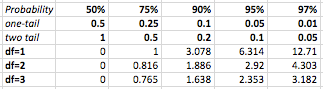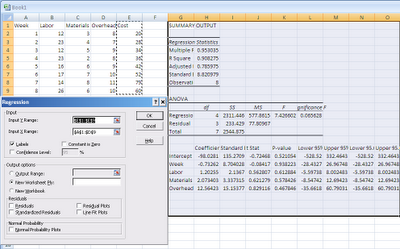## Pages

### The Numbers in a Business Trend22 June, 2010

Statistics is a subject taught, and usually required in business schools. What’s the reason? It helps us see not only the trends in Business, but what parts of those trends are statistically significant. Regression analysis lets us see what matters in determining costs and prices, and is usually run in conjunction with historical data. In order to explain regression analysis, we need to define a few things:

Median: The middle value in an ordered set of numbers that separates the lower half from the higher half.

Mean: The numeric average of a set of numbers.

Mode: The numeric value in a set of numbers that occurs most frequently.

Variance: The dispersion of numbers in a set around the mean.

Standard Deviation: The variance of a set of numbers whose unit of measurement is the same as those of the number set.

CV (Coefficient of Variation): A ratio of standard deviation to the mean.

R2 (Coefficient of Determination): The ratio of explained variance to total variance.

Standard Error: A measure of fluctuation from one sample statistic to another.

Normal Distribution (standard normal): A bell curved graph used to describe number sets with complete data or a high sample size.

Student’s Distribution (t-distribution): A bell curved graph used to describe number sets when the sample size is small.

Manual Regression: Using a number set to generate a line equation that resembles the trend of the data.

T-Score: An indication of correctness of a hypothesis. This is sometimes called the t-stat.

P-Value: A value that shows the significance of a factor.

Degrees of Freedom: The variability in the curve of a statistic. Generally this equals n-(k+1) where n is the sample size and k is the number of independent variables.

T-Table: A lookup table where one axis represents degrees of freedom and the other axis represents the probability. A T-Score is found where the correct column and row intersect.Hypothesis Testing: First, you must choose both a hypothesis and an inverse of the hypothesis, such that you can prove one of them by choosing the correct probability when using the T-Table. A null hypothesis (the opposite of what you want to prove) and an alternative hypothesis (what you want to prove) are chosen as equality equations. The equality of the null and alternative hypotheses determines what kind of tail test to use in the lookup table. If the equality symbol used in the alternative hypothesis is a '>', it is a right tailed test. If it is a '<', you have a left-tailed test. If it is neither, you have a two-tailed test. A null hypothesis is generally used for the test because of historical significance in the philosophy of math as well as the innocent until proven guilty axiom.

The following example deals with salaries. If a study claims that programmers make \$100,000/year, but you think they make more, H1(The alternative hypothesis) is x > 100,000. The null hypothesis would then be H0(the notation for a null hypothesis): x <= 100,000. The alternative and null hypothesizes are always compliments (opposites) of each other, and the alternative hypothesis (what you are trying to prove) should be stated first.

After comparing data sets using the equations that follow, and comparing the t-stat to the critical value found in the T-Table lookup, we'll have enough information to determine what is correct. Using the standard deviation, t-score, and our data set, we have enough data to make a conclusion.

The significance of the right, left, or two tailed choice is that anything that falls respectively to the right, left or both of the critical regions denoted by the T-Table lookup number equates to rejecting H0, and accepting H1.

Equations:That's the theory. Most people will simply use Excel or some other program to run all the calculations automatically. However, understanding what is going on, and how to correctly interpret what Excel tells you is much easier if you understand that theory.

Using Excel’s Data Analysis package, you can select columns to include in a regression, at which point you’ll get a nice little table like the following:So, what does this mean? Well, the point of a regression analysis is to create a fitted line to the scattered data points that we have. The key here is that we want to find a relationship between x (the numbers that drive our end result) and y (our end result) in our plots. The bottom line is: the more significant the variables are, the bigger the slope of the line will be. This also means that the if the relationship is small, the slope of the line will be small. We could say that the closer to 0 the slope is, the less significant that slope is. Because we are trying to prove that something is significant and that the slope is not 0, we can use the following hypothesis. H0: x =0, H1: x!=0, where x is the slope of the regression line. We can take our t-statistic (t) equation from above and see if the slope is statistically significant.

Let's use an example that uses Excel's linear regression to preform a hypothesis test. Using a setup as shown in the screenshot above, Excel gives us a t-stat. We must now use a t-table (google it if you don't have a complete one) to compare the t-stat given by excel to what probability we want. Usually 95% is the standard probability used. Our df (degrees of freedom) is 3. We get this from using the df definition given above, and the data from the screenshot (showing an example of material cost effect on overall cost) : n-(k+1) -> 8-(4+1) -> 3. The T-Table score is 2.353. Excel says our t-stat is .62 for materials. Because .62 is not > 2.353, we do not reject H0, and conclude that the material cost is not significant in the overall cost!

A couple of important notes now that we have seen how hypothesis testing works:
• Rejection does not mean something is false, it means that it is not reasonable.
• Accepting does not mean something is true, it means it is reasonable.
• Some people use a p-value rule: Reject H0 if p <>
• Don't just believe the output. Always make sure the numbers make sense.